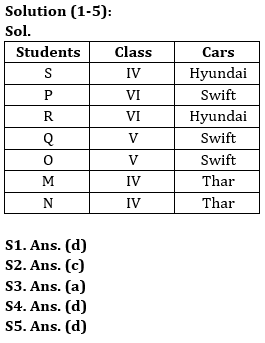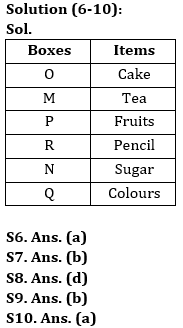Latest Banking jobs   »

# Reasoning Quizzes Quiz For IBPS RRB PO/Clerk Prelims 2023-23rd May

Directions (1-5): Answer the questions based on the information given below.
Seven students M, N, O, P, Q, R and S study in three classes (IV, V and Vl) and they like three type of cars (Swift, Thar, Hyundai) such that at least two student study in the same class and at least two students like the same type of car. All the information given is not in the same manner.
Only Q studies in the same class as O and both of them like the same car. S study in class IV and likes Hyundai. Two students who study in class IV like Thar. P study in class Vl and likes Swift. Only R studies in the same class as P and likes Hyundai.

Q1. Who among the following student study in class IV?
(a) M
(b) N
(c) R
(d) Both M and N
(e) None of these

Q2. Who among the following student likes Swift?
(a) M
(b) N
(c) Q
(d) Either N or Q
(e) None of these

Q3. N studies in which of the following class?
(a) Class IV
(b) Class V
(c) Class VI
(d) Either IV or VI
(e) None of these

Q4. Which of the following combination of students represents the students who like Swift?
(a) P, M, O
(b) P, N, Q
(c) P, M, N
(d) Q, O, P
(e) None of these

Q5. Choose the incorrect combination of student – class – car from the given alternatives.
(a) Q – Class V – Swift
(b) M – Class IV – Thar
(c) N – Class IV – Thar
(d) M – Class IV – Hyundai
(e) O – Class V – Swift

Directions (6-10): Answer the questions based on the information given below.
Six boxes M, N, O, P, Q and R contain different items and are kept one above the other from bottom to top (but not in the same order as given). The bottommost box is numbered as 1 and the topmost box is numbered as 6.
M is immediately above the box containing Fruits. There are two boxes between Q and the box containing Fruits. R is just above the box containing Sugar. R does not contain fruits. Neither M nor Q contains Sugar. O contains cake and is above P which is above the box containing colours. N is just above the box containing colours. Box containing Tea is above the box containing Pencil.

Q6. Which among the following box is at topmost position?
(a) O
(b) R
(c) N
(d) M
(e) None of these

Q7. Which of the following box is immediately below P?
(a) N
(b) R
(c) M
(d) Q
(e) None of these

Q8. How many boxes are below O?
(a) Three
(b) Four
(c) One
(d) Five
(e) None of these

Q9. Which of the following statement(s) is/are true?
I. N is above O
II. P contains fruits
III. Box containing tea is kept just below O
(a) Only II
(b) Only II and III
(c) Only III
(d) Only I
(e) Only I and III

Q10. Which of the following box is immediately above Q?
(a) Box containing sugar
(b) Box containing tea
(c) Box containing pencil
(d) Box containing colour
(e) None of the above

Solutions## FAQs

#### Congratulations!Union Budget 2023-24: Free PDF